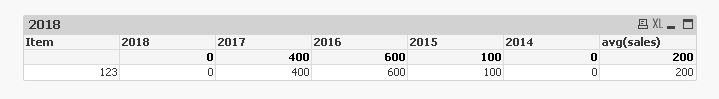# New to QlikView

Discussion board where members can get started with QlikView.

Announcements
HighlightedPartner

## Help with Average but ignore zero

Hi all,

I have some expressions that give sales by year. i have some years that are zero and i am trying to ignore those zeros when using avg().

example..

item 123 has sales in 2013, 2015, 2016 and 2017 but no sales in 2014 and 2018

i have a straight table that has the last 5 years sales (so i am not including 2013).The AVG() is taking the full sales values (including 2013) and averaging it across all years, but what i actually want it to do is to only sum the years with values that i have in the chart (2015, 2016, 2017) and divide that total by 3 (as only 3 of the years have values)

so in this example i am trying to get 366.6

Can anyone help?

sample attached.

1 Solution

Accepted Solutions
HighlightedMVP

## Re: Help with Average but ignore zero

May be this

Sum({<Year={\$(=Max(Year)), \$(=Max(Year)-1), \$(=Max(Year)-2), \$(=Max(Year)-3), \$(=Max(Year)-4)}>}sales)/

Count(DISTINCT {<sales = {">0"}, Year={\$(=Max(Year)), \$(=Max(Year)-1), \$(=Max(Year)-2), \$(=Max(Year)-3), \$(=Max(Year)-4)}>} Year)

or this

Avg({<Year={\$(=Max(Year)), \$(=Max(Year)-1), \$(=Max(Year)-2), \$(=Max(Year)-3), \$(=Max(Year)-4)}, sales = {">0"}>}sales)

3 Replies
HighlightedMVP

## Re: Help with Average but ignore zero

May be this

Sum({<Year={\$(=Max(Year)), \$(=Max(Year)-1), \$(=Max(Year)-2), \$(=Max(Year)-3), \$(=Max(Year)-4)}>}sales)/

Count(DISTINCT {<sales = {">0"}, Year={\$(=Max(Year)), \$(=Max(Year)-1), \$(=Max(Year)-2), \$(=Max(Year)-3), \$(=Max(Year)-4)}>} Year)

or this

Avg({<Year={\$(=Max(Year)), \$(=Max(Year)-1), \$(=Max(Year)-2), \$(=Max(Year)-3), \$(=Max(Year)-4)}, sales = {">0"}>}sales)

Highlighted
Contributor II

## Re: Help with Average but ignore zero

Hi Chris,

The following expression also should works:

Avg({<Year = {">=\$(=max(Year)-4)"}, sales = {">0"}>}sales)

Thanks,

Vitalii

HighlightedPartner

## Re: Help with Average but ignore zero

Thats great, thanks Sunny, i have used the first solution you gave. the second one gave different results in my real data to the first for some reason.